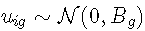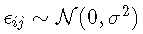# 数据集

## 子样本

`head(data)`

`summary(data)`

## 简易智能量表评分结果

``` hist( MMSE )

hist( norm )```

# R语言如何用潜类别混合效应模型（lcmm）分析抑郁症状

```color <-ID

xyplot```

## 考虑的模型和## 因变量：归一化 简易智能量表评分

`normMMSE <- norm`

## 估计只有一个类的模型 (G=1)

```

age65 <- (age - 65)/10```

```
lme(norm ~ age65+I(age65^2)+CEP rand =~ age65+I(age65^2) subject = 'ID'```

# 从通过假设单个潜在类估计的模型，我们现在可以搜索异构概况。下一行提供了使用  G>1 时初始值对 2 个潜在类的模型的估计。

```

#考虑到2类的估计
lme(ng = 2, mix=~age65+I(age65^2))```

## 初始值

### 用户预先指定的值

`lme( B = c(0, 50, 30, 3, -1))`

# 另一种方法是从 1 类模型的估计值的渐近分布中随机生成初始值（此处为 `m1`）：

### 随机生成的值

`lme(rand(m1))`

### 网格搜索

`grid(lme iter=30,)`

## 选择最佳模型

`summary`

```

summary(m2d)```

# 模型的预测

`data.frame(age=seq(65,95,l=50))`

`predictY`

```plot(prd0)

`plot(IC,, shades=TRUE)`

```par(mfrow=c(1,3))

plot(pr1 )

plot(pr0

plot(pr3)```

## 最终潜在类混合模型的评估

```

plot(m)```

### 预测与观察的图表

`plot(m, shad = TRUE)`

# 分类

`postprob(m2d)`

`Class 1` 由 62 个样本 (12.4%) 组成，而 438 个样本属于第二类。

• 后验分类表：分类在 `class 1` (resp.  `class 2`) 中的对象属于该类的平均概率为 0.8054 (resp. 0.8730)。这显示了类别的良好区分。
• 高于阈值的分类的比例：这里 90.18%（分别为 61.29%）的第 1 类（分别为 2）的后验概率大于 70%。

Kaizong Ye拓端研究室（TRL）的研究员。

​非常感谢您阅读本文，如需帮助请联系我们！QQ在线咨询

15121130882

0571-63341498

## 关注有关新文章的微信公众号

This will close in 0 seconds